# Adding Fractions with Unlike Denominators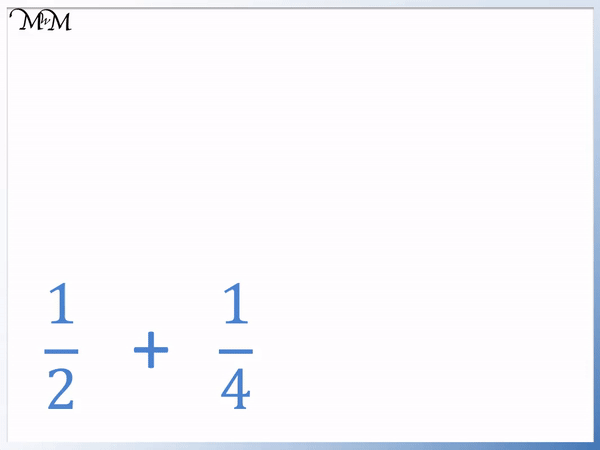•   1 / 2   has a of ‘2’ and   1 / 4   has a of ‘4’.
• Fractions that have different denominators are called unlike frations.
• We can see that   1 / 2   is the same as   2 / 4 .
• Like fractions are fractions that have the same .
• We can add like fractions by adding the together and keeping the denominator the same.
• We count the number of that we have in total.
• ‘2’ quarters plus ‘1’ quarter makes ‘3’ quarters.
We can add like fractions because they have a common denominator.

This common denominator remains the same in the answer and then add the numerators together.•   1 / 2  and   1 / 4  are unlike fractions because they have different .
• We write 1 / 2  as an that has the same denominator as 1 / 4 .
• To make 1 / 2 have a denominator of ‘4’, we double the denominator.
• Since we have doubled the denominator, we must also double the numerator.
• 1 / 2 is the same as 2 / 4.
• Now we have like fractions, we can add them by keeping the denominator the same and adding the numerators.
• The denominator of the answer will remain as ‘4’.
• 2 + 1 = 3.
• 1 / 2 + 1 / 4   = 3 / 4•   1 / 12  and   3 / 4  are unlike fractions because they have different .
• Since ‘4’ is a of ’12’, we will write the fractions out of ’12’.
• To make 3 / 4 have a denominator of ’12’, we multiply the denominator by 3.
• Since we have multiplied the denominator by 3, we must also multiply the numerator by 3.
• 3 / 4 is the same as 9 / 12.
• Now we have like fractions, we can add them by keeping the denominator the same and adding the numerators.
• The denominator of the answer will remain as ’12’.
• 1 + 9 = 10.
• 1 / 12 + 3 / 4   = 10 / 12•   1 / 2  and   1 / 5  are unlike fractions because they have different .
• The denominators of ‘2’ and ‘5’ do not divide into each other and so we need to find another common denominator that they both divide into.
• To find the common denominator, list the times tables of both denominators and the lowest common denominator is the first number to appear in both lists.
• 10 is the first number that is in both the ‘2’ and the ‘5’ times table and so, we will write both fractions out of ’10’.
• By multiplying both the numerator and denominator by 5, we can write   1 / 2 as 5 / 10.
• By multiplying both the numerator and denominator by 2, we can write   1 / 5 as 2 / 10.
• Both fractions are now written as like fractions and can be added.
• The denominator of the answer will remain as ’10’.
• 5 + 2 = 7.
• 1 / 2 + 1 / 5   =   7 / 10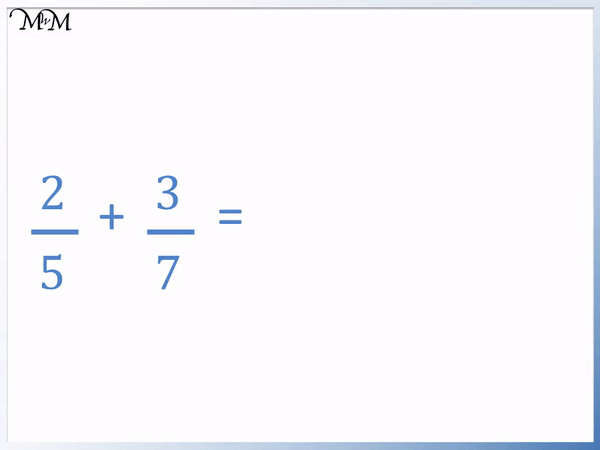• This is an example of using the ‘butterfly method’ to add fractions.
• The denominator is found by multiplying the two denominators together.
• We multipy across each diagonal as shown.
• 2 x 7 = 14.
• 3 x 5 = 15.
• We add these results to obtain our numerator of 29.
• 2 / 5 + 3 / 7   =   29 / 35Supporting Lessons

# How to Add Unlike Fractions by Only Changing One Fraction

The numerator of a fraction is the number on the top of a fraction, above the dividing line.

The denominator of a fraction is the number on the bottom of a fraction, below the dividing line.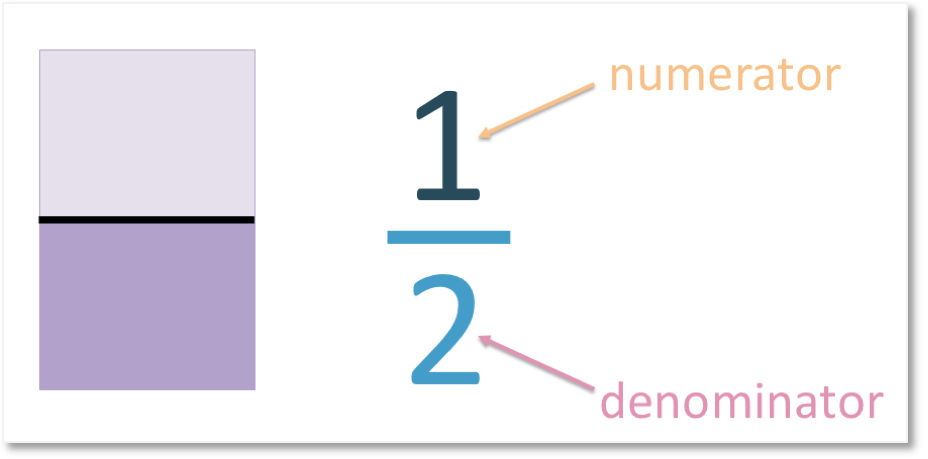Like fractions are two or more fractions that have the same denominator.

Unlike fractions are fractions that do not have the same denominator. The denominators are different numbers.

In this lesson we look at the steps required to add fractions with unlike denominators by converting the fractions into like fractions.

The steps to add fractions with unlike denominators are:

1. Find the lowest common denominator, which is the first number to appear in both times tables of the given denominators.
2. Rewrite each fraction as an equivalent fraction containing this common denominator by multiplying its numerator and denominator by the same value.
3. The fractions are now like fractions and can be added by keeping the denominator the same and adding the numerators.

In order to better understand these steps, we will look at some examples.

We will start by looking at examples where only one fraction will change.

12   +   14 .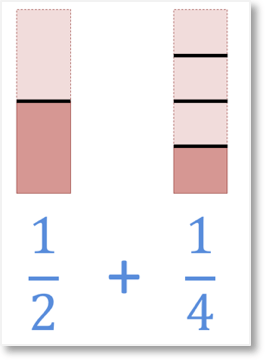However, this is not easy to calculate because the fractions are divided into different sizes. Therefore the two fractions are unlike fractions.

This is because the denominators are different and the denominator tells us how many equal parts to divide the shape into.

The shapes have been divided into a different number of parts. To be able to add these two fractions, the parts need to be the same size so we can count them.

The denominators need to be the same. When fractions have the same denominator we say that they have a common denominator.Here we have divided the first shape so that it also has four equal parts. The same proportion of the shape is still shaded in. However, the total number of parts has changed.

Now that the parts are the same size, we can add the fractions.We can see that if we combine the two-quarters with the one-quarter that we have a total of three-quarters.

24   +    14    =    34.

Therefore,

12    +    14    =     34.

Once two fractions have the same denominator, we can simply add the numerators and keep the denominator the same. To change a fraction so that it has the same denominator as another fraction, we need to find their lowest common multiple.

This means, the smallest number that both denominators divide into.

We will use our previous example:We are looking for the smallest number that both 2 and 4 divide into. We can start by looking at the largest denominator and seeing if both numbers divide into it. The largest denominator out of the two fractions is the 4.

Both 2 and 4 divide into 4 since 2 x 2 = 4. So, the smallest number that both denominators divide into is 4.We will change one fraction so that they are both quarters i.e. both have a denominator of 4.

To find a fraction that is the same size, but has a different numerator and denominator, we are finding an equivalent fraction.

We must change both the numerator and the denominator by the same proportion. To do this, we must multiplyOne-quarter already has a denominator of 4, so we don’t need to change it. We can keep this fraction the same.

However, one-half has a different denominator of 2.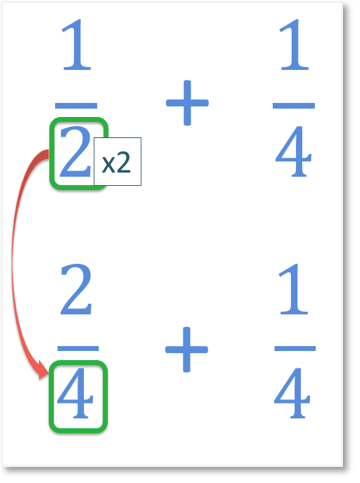To work out what the numerator will be, we must find out what we multiply the denominator by to get to 4. To get from 2 to 4, we multiply by 2.Because we multiplied the denominator by 2, we must also multiply the numerator by 2.

1 x 2 = 2

The numerator is therefore 2.

We now have

24   +   14.

We now have two like fractions which have the same denominator.

To add like fractions, we keep the denominator the same and we add the numerators.

2 + 1 = 3

Therefore,

24   +   14    =    34

and so,

12   +    14     =   34.

# How to Add Unlike Fractions by Changing Both Fractions

In the example above it was only necessary to change one fraction before adding them.

This was because one fraction’s denominator was a factor of the other denominator. This means that the smaller denominator could be multiplied by a number to make the larger denominator.

Now we will look at examples in which this is not the case. We will be changing both fractions in order to obtain a common denominator and make them like fractions.

We want to find the smallest denominator to keep the fractions as simple as possible. This is known as the lowest common denominator.

To find the lowest common denominator use the following steps:

• List the multiples (times tables) of each of the given denominators.
• The lowest common denominator is the first number to appear in both lists.
• If the denominators cannot both be divided by the same number then the lowest common denominator is equal to the denominators multiplied together.

Here is an example of adding fractions with unlike denominators.

12   +   15 .To be able to add these two unlike fractions, the denominators must first be the same. We begin by finding the lowest common denominator.

This is the smallest number that both denominators: 2 and 5 divide into.To find the lowest common denominator, we list the first few numbers in each times table, and continue until we find a number in both lists.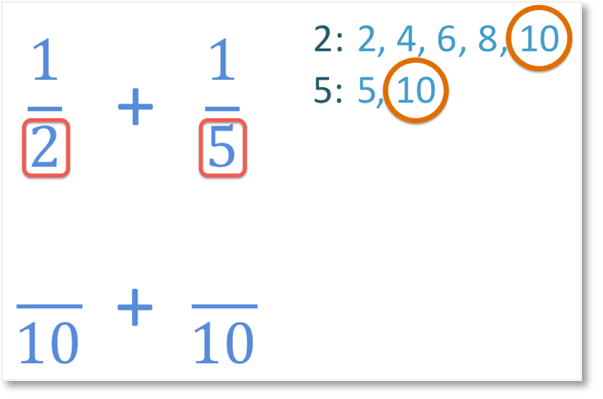The smallest number in both the 2 and 5 times tables is 10. So, 10 will be our lowest common denominator.

Note that it would have been possible to multiply the denominators of 2 and 5 to make the common denominator of 10 in this example.

This is because there is not a whole number that can divide into both 2 and 5 exactly.

Because we have changed the denominators of both fractions, we must also change their numerators. We must change the numerator and denominator of each fraction in proportion, so we multiply.

We follow the rule for finding equivalent fractions: whatever we multiply the denominator by, we must multiply the numerator by.

So, we begin by finding out what we multiplied each denominator by to get to 10.To get from 2 to our new denominator of 10, we multiplied by 5.So we must also multiply the numerator by 5.

1 x 5 = 5The numerator is therefore 5.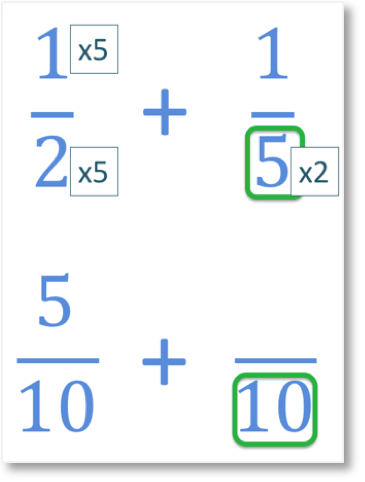To get from 5 to 10, we multiplied by 2.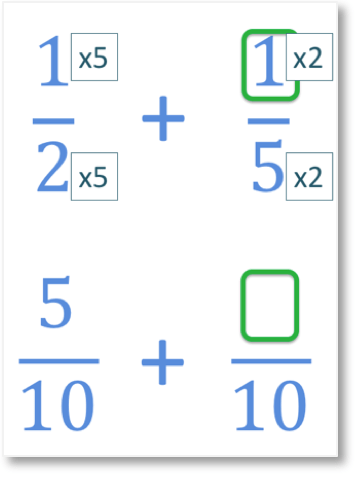So we must also multiply the numerator by 2.

1 x 2 = 2The numerator is therefore 2.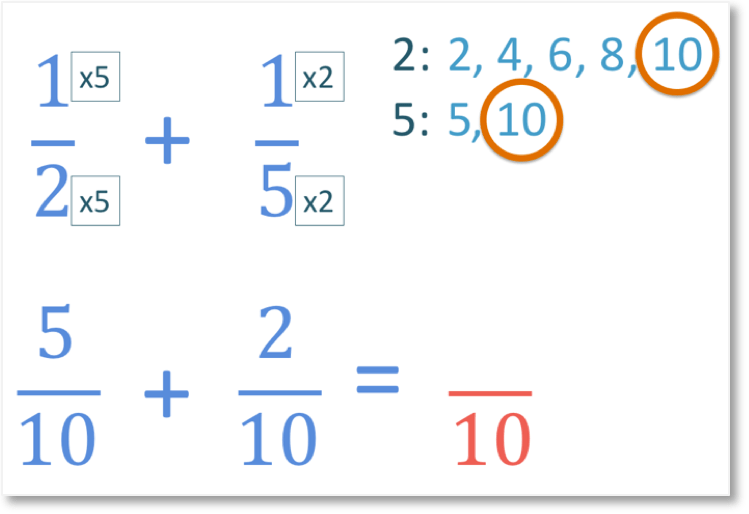Both denominators are now the same, so we have two like fractions and we can add them.

To add like fractions, we add the numerators but keep the denominator the same as the common denominator.We can now add the numerators.

5 + 2 = 7

The numerator is therefore 7. And so,

510   +   210   =   710

Therefore,

12   +   15   =   710

When teaching adding fractions with unlike denominators, the most common error is to add both the numerators and the denominators together without finding a common denominator.

To avoid this very common mistake, refer back to visual models such as the bar models shown above and keep reminding them that we must find a common denominator before adding.

It is also common for some students to always multiply the two given denominators together when finding the lowest common denominator.

Whilst this will always find a common denominator, it will not give the lowest common denominator in some circumstances where the denominators share common factor.

This may result in them obtaining a larger answer through larger multiplications that needs simplifying at the end.

# How to Add Unlike Fractions using the ‘Butterfly Method’

A methodical procedure known as the ‘Butterfly Method’ for adding fractions is shown below. I would not recommend introducing the topic of adding fractions with the method below but it can provide an easier structure to some people and help with mental addition of the fractions.In this example, we are adding 25   +   37.

These fractions have unlike denominators and both 5 and 7 do not have a shared factor. This means that there is not a whole number (apart from 1) that can divide exactly into 5 and 7.

The denominator of the answer will be the two denominators multiplied together.

5 x 7 = 35

We can multiply across the diagonals and then add these answers together to obtain the numerator.

2 x 7 = 14

and

3 x 5 = 15

14 + 15 = 29

and so, 29 is the numerator of our answer.

25   +   37   =   2935  .Now try our lesson on Subtracting Fractions with a Common Denominator where we learn how to subtract like fractions.